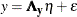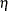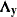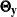### The Measurement Model for y

The first component of the LISMOD specification is the measurement model for y, as shown in the following equation:In the context of covariance structure analysis, without loss of generality, it is assumed that y andare centered so that there is no intercept term in the equation. This equation essentially states that y is a function of the true scores vectorplus the error term, which is independent of. The model matrices involved in this measurement model are(effects ofon y) and, which is the covariance matrix of.

For the career aspiration data, you specify the following two MATRIX statements for this measurement model:

   matrix _lambday_ [1,1], [2,1] = 1.0, [3,2] = 1.0, [4,2];
matrix _thetay_  [4,1], [3,2];


The first matrix statement is for matrix. You specify four parameters in this matrix. The [1,1] and [4,2] elements are free parameters, and the [2,1] and [3,2] elements have fixed values of 1. You do not specify other elements in this matrix. By default, unspecified elements in thematrix are fixed zeros. You can check your initial model specification of this matrix, as shown in the Figure 17.47.

Figure 17.47: Career Aspiration Analysis 3: Initial Measurement Model for y

Initial _LAMBDAY_ Matrix
R_Amb F_Amb
rea
 . [_Parm01]
 0
roa
 1.0000
 0
foa
 0
 1.0000
fea
 0
 . [_Parm02]

Initial _THETAY_ Matrix
rea roa foa fea
rea
 0
 0
 . [_Parm13]
roa
 0
 . [_Parm14]
 0
foa
 0
 . [_Parm14]
In Figure 17.47, the initial _LAMBDAY_ matrix is a 42 matrix. The _LAMBDAY_ matrix contains information about the relationships between the row indicator variables y (YVAR= variables) and the column factors(ETA= variables). As specified in the MATRIX statement for _LAMBDAY_, the [1,1] and [4,2] are free parameters named _Parm01 and _Parm02, respectively. These parameter names are generated by PROC CALIS. Fixed values 1.0 appear in the [2,1] and [3,2] elements. These fixed values are used to identify the scales of the latent variables R_Amb and F_Amb.
The _THETAY_ matrix in Figure 17.47 is the covariance matrix among the error terms for the y-variables (YVAR= variables). This is a 44 matrix for the four measured indicators. As specified in the MATRIX statement for _THETAY_, the [4,1] and [3,2] elements are free parameters named _Parm13 and _Parm14, respectively. Because _THETAY_ is a symmetric matrix, elements [1,4] and [2,3] are also implicitly specified as parameters in this model matrix.
As shown in Figure 17.47, PROC CALIS adds four default free parameters to the _THETAY_ matrix. On the diagonal of the _THETAY_ matrix, parameters _Add07, _Add08, _Add09, and _Add10 are added as default free parameters by PROC CALIS automatically. In general, error variances are default free parameters in PROC CALIS. You do not have to specify them but you can specify them if you want to, especially when you need to set fixed values or other constraints on them.